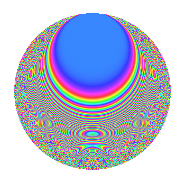# Properties

 Label 4033.2.a.fLevel 4033 Weight 2 Character orbit 4033.a Self dual Yes Analytic conductor 32.204 Analytic rank 0 Dimension 85 CM No

# Learn more about

## Newspace parameters

 Level: $$N$$ = $$4033 = 37 \cdot 109$$ Weight: $$k$$ = $$2$$ Character orbit: $$[\chi]$$ = 4033.a (trivial)

## Newform invariants

 Self dual: Yes Analytic conductor: $$32.2036671352$$ Analytic rank: $$0$$ Dimension: $$85$$ Fricke sign: $$-1$$ Sato-Tate group: $\mathrm{SU}(2)$

## $q$-expansion

The dimension is sufficiently large that we do not compute an algebraic $$q$$-expansion, but we have computed the trace expansion.

 $$\operatorname{Tr}(f)(q) =$$ $$85q$$ $$\mathstrut +\mathstrut 11q^{2}$$ $$\mathstrut +\mathstrut 21q^{3}$$ $$\mathstrut +\mathstrut 93q^{4}$$ $$\mathstrut +\mathstrut 12q^{5}$$ $$\mathstrut +\mathstrut 4q^{6}$$ $$\mathstrut +\mathstrut 17q^{7}$$ $$\mathstrut +\mathstrut 30q^{8}$$ $$\mathstrut +\mathstrut 98q^{9}$$ $$\mathstrut +\mathstrut O(q^{10})$$ $$\operatorname{Tr}(f)(q) =$$ $$85q$$ $$\mathstrut +\mathstrut 11q^{2}$$ $$\mathstrut +\mathstrut 21q^{3}$$ $$\mathstrut +\mathstrut 93q^{4}$$ $$\mathstrut +\mathstrut 12q^{5}$$ $$\mathstrut +\mathstrut 4q^{6}$$ $$\mathstrut +\mathstrut 17q^{7}$$ $$\mathstrut +\mathstrut 30q^{8}$$ $$\mathstrut +\mathstrut 98q^{9}$$ $$\mathstrut +\mathstrut 9q^{10}$$ $$\mathstrut +\mathstrut 37q^{11}$$ $$\mathstrut +\mathstrut 44q^{12}$$ $$\mathstrut +\mathstrut 14q^{13}$$ $$\mathstrut +\mathstrut 26q^{14}$$ $$\mathstrut +\mathstrut 27q^{15}$$ $$\mathstrut +\mathstrut 85q^{16}$$ $$\mathstrut +\mathstrut 34q^{17}$$ $$\mathstrut +\mathstrut 3q^{18}$$ $$\mathstrut +\mathstrut 15q^{19}$$ $$\mathstrut +\mathstrut 15q^{20}$$ $$\mathstrut +\mathstrut 17q^{21}$$ $$\mathstrut +\mathstrut q^{22}$$ $$\mathstrut +\mathstrut 72q^{23}$$ $$\mathstrut +\mathstrut 15q^{24}$$ $$\mathstrut +\mathstrut 85q^{25}$$ $$\mathstrut +\mathstrut 33q^{26}$$ $$\mathstrut +\mathstrut 69q^{27}$$ $$\mathstrut +\mathstrut 7q^{28}$$ $$\mathstrut +\mathstrut 19q^{29}$$ $$\mathstrut -\mathstrut 9q^{30}$$ $$\mathstrut +\mathstrut 23q^{31}$$ $$\mathstrut +\mathstrut 51q^{32}$$ $$\mathstrut +\mathstrut 32q^{33}$$ $$\mathstrut +\mathstrut 49q^{34}$$ $$\mathstrut +\mathstrut 40q^{35}$$ $$\mathstrut +\mathstrut 121q^{36}$$ $$\mathstrut +\mathstrut 85q^{37}$$ $$\mathstrut +\mathstrut 84q^{38}$$ $$\mathstrut +\mathstrut 39q^{39}$$ $$\mathstrut +\mathstrut 22q^{40}$$ $$\mathstrut +\mathstrut 55q^{41}$$ $$\mathstrut -\mathstrut 28q^{42}$$ $$\mathstrut +\mathstrut 78q^{44}$$ $$\mathstrut +\mathstrut 28q^{45}$$ $$\mathstrut +\mathstrut 17q^{46}$$ $$\mathstrut +\mathstrut 184q^{47}$$ $$\mathstrut +\mathstrut 97q^{48}$$ $$\mathstrut +\mathstrut 88q^{49}$$ $$\mathstrut +\mathstrut 26q^{50}$$ $$\mathstrut +\mathstrut 27q^{51}$$ $$\mathstrut +\mathstrut 73q^{52}$$ $$\mathstrut +\mathstrut 64q^{53}$$ $$\mathstrut +\mathstrut 31q^{54}$$ $$\mathstrut +\mathstrut 39q^{55}$$ $$\mathstrut +\mathstrut 68q^{56}$$ $$\mathstrut -\mathstrut 33q^{57}$$ $$\mathstrut +\mathstrut 28q^{58}$$ $$\mathstrut +\mathstrut 60q^{59}$$ $$\mathstrut -\mathstrut 22q^{60}$$ $$\mathstrut +\mathstrut 7q^{61}$$ $$\mathstrut +\mathstrut 70q^{62}$$ $$\mathstrut +\mathstrut 28q^{63}$$ $$\mathstrut +\mathstrut 102q^{64}$$ $$\mathstrut +\mathstrut 17q^{65}$$ $$\mathstrut -\mathstrut 15q^{66}$$ $$\mathstrut +\mathstrut 82q^{67}$$ $$\mathstrut +\mathstrut 92q^{68}$$ $$\mathstrut +\mathstrut 22q^{69}$$ $$\mathstrut -\mathstrut 41q^{70}$$ $$\mathstrut +\mathstrut 113q^{71}$$ $$\mathstrut -\mathstrut 19q^{73}$$ $$\mathstrut +\mathstrut 11q^{74}$$ $$\mathstrut +\mathstrut 45q^{75}$$ $$\mathstrut +\mathstrut 34q^{76}$$ $$\mathstrut +\mathstrut 64q^{77}$$ $$\mathstrut +\mathstrut 29q^{78}$$ $$\mathstrut +\mathstrut 23q^{79}$$ $$\mathstrut +\mathstrut 54q^{80}$$ $$\mathstrut +\mathstrut 149q^{81}$$ $$\mathstrut +\mathstrut 4q^{82}$$ $$\mathstrut +\mathstrut 100q^{83}$$ $$\mathstrut -\mathstrut 49q^{84}$$ $$\mathstrut -\mathstrut 5q^{85}$$ $$\mathstrut -\mathstrut 24q^{86}$$ $$\mathstrut +\mathstrut 65q^{87}$$ $$\mathstrut +\mathstrut 14q^{88}$$ $$\mathstrut +\mathstrut 84q^{89}$$ $$\mathstrut -\mathstrut 21q^{90}$$ $$\mathstrut +\mathstrut 32q^{91}$$ $$\mathstrut +\mathstrut 95q^{92}$$ $$\mathstrut +\mathstrut 19q^{93}$$ $$\mathstrut -\mathstrut 47q^{94}$$ $$\mathstrut +\mathstrut 102q^{95}$$ $$\mathstrut +\mathstrut 29q^{96}$$ $$\mathstrut +\mathstrut 7q^{97}$$ $$\mathstrut +\mathstrut 26q^{98}$$ $$\mathstrut +\mathstrut 107q^{99}$$ $$\mathstrut +\mathstrut O(q^{100})$$

## Embeddings

For each embedding $$\iota_m$$ of the coefficient field, the values $$\iota_m(a_n)$$ are shown below.

For more information on an embedded modular form you can click on its label.

Label $$a_{2}$$ $$a_{3}$$ $$a_{4}$$ $$a_{5}$$ $$a_{6}$$ $$a_{7}$$ $$a_{8}$$ $$a_{9}$$ $$a_{10}$$
1.1 −2.76864 3.12213 5.66539 −1.61337 −8.64407 −3.34335 −10.1482 6.74771 4.46684
1.2 −2.68964 −0.345637 5.23416 3.86855 0.929638 −2.16399 −8.69874 −2.88054 −10.4050
1.3 −2.66672 −1.32046 5.11137 0.282486 3.52128 −2.11170 −8.29715 −1.25639 −0.753309
1.4 −2.62495 2.49737 4.89034 0.915854 −6.55547 2.77253 −7.58698 3.23688 −2.40407
1.5 −2.49324 −1.70202 4.21624 −1.58478 4.24353 2.99306 −5.52560 −0.103143 3.95124
1.6 −2.42751 0.768452 3.89281 −1.80408 −1.86543 −1.49541 −4.59481 −2.40948 4.37943
1.7 −2.36110 2.47312 3.57478 1.50101 −5.83928 4.30200 −3.71820 3.11634 −3.54403
1.8 −2.31084 0.814794 3.33999 −0.287417 −1.88286 −2.20640 −3.09651 −2.33611 0.664174
1.9 −2.27621 −2.60153 3.18111 2.30412 5.92161 1.78008 −2.68845 3.76795 −5.24464
1.10 −2.26706 −2.69699 3.13957 −3.90758 6.11425 −4.79951 −2.58348 4.27377 8.85872
1.11 −2.15921 −0.135675 2.66221 −3.14294 0.292951 0.215452 −1.42985 −2.98159 6.78628
1.12 −2.11247 2.70421 2.46253 2.42834 −5.71257 1.36575 −0.977087 4.31276 −5.12979
1.13 −2.03156 3.25300 2.12723 1.52354 −6.60865 −4.26037 −0.258468 7.58200 −3.09515
1.14 −1.99373 −2.36120 1.97494 −0.776635 4.70759 −0.440394 0.0499623 2.57527 1.54840
1.15 −1.96262 −1.03474 1.85187 3.48986 2.03080 −2.58926 0.290726 −1.92931 −6.84926
1.16 −1.92648 −3.23973 1.71134 1.34988 6.24129 3.96616 0.556094 7.49587 −2.60052
1.17 −1.88550 3.14155 1.55513 −2.47668 −5.92341 4.66231 0.838810 6.86935 4.66980
1.18 −1.82466 0.0328358 1.32938 −0.181740 −0.0599140 4.41961 1.22366 −2.99892 0.331613
1.19 −1.67574 1.31893 0.808091 −4.46158 −2.21019 0.153470 1.99732 −1.26041 7.47643
1.20 −1.66354 1.93600 0.767368 −2.27919 −3.22061 0.280246 2.05053 0.748091 3.79153
See all 85 embeddings
 $$n$$: e.g. 2-40 or 990-1000 Embeddings: e.g. 1-3 or 1.85 Significant digits: Format: Complex embeddings Normalized embeddings Satake parameters Satake angles

## Inner twists

This newform does not have CM; other inner twists have not been computed.

## Atkin-Lehner signs

$$p$$ Sign
$$37$$ $$-1$$
$$109$$ $$1$$

## Hecke kernels

This newform can be constructed as the kernel of the linear operator $$T_{2}^{85} - \cdots$$ acting on $$S_{2}^{\mathrm{new}}(\Gamma_0(4033))$$.